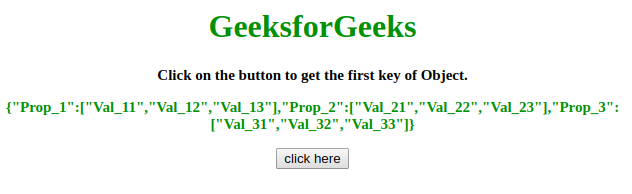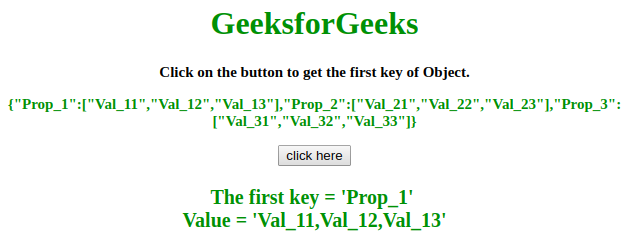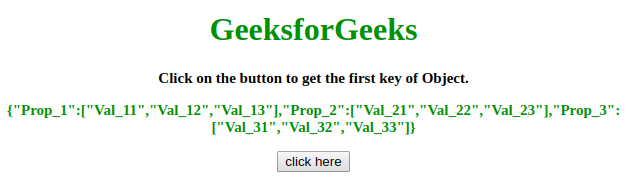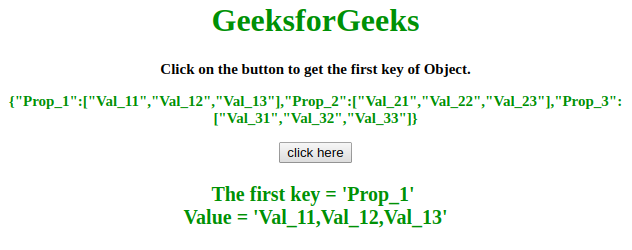# How to get the first key name of a JavaScript object ?

Given an object and the task is to get the first key of a JavaScript Object. Since JavaScript object does not contains numbered index so we use the following approaches to get the first key name of the object.

Approach 1:

• First take the JavaScript Object in a variable.
• Use object.keys(objectName) method to get access to all the keys of object.
• Now, we can use indexing like Object.keys(objectName) to get the key of first element of object.

Example: This example illustrate the above approach.

 `  ` `<``html``>  ` ` `  `<``head``>  ` `    ``<``title``>  ` `        ``How to get the first key name ` `        ``of a JavaScript object ? ` `    ``      ` `  ` ` `  `<``body` `style` `= ``"text-align:center;"``>  ` `     `  `    ``<``h1` `style` `= ``"color:green;"` `>  ` `        ``GeeksforGeeks  ` `    ``  ` `     `  `    ``<``p` `id` `= ``"GFG_UP1"` `style` `=  ` `        ``"font-size: 15px; font-weight: bold;"``>  ` `    `` ` `     `  `    ``<``p` `id` `= ``"GFG_UP2"` `style` `=  ` `        ``"font-size: 15px; font-weight: bold; color: green;"``>  ` `    `` ` `     `  `    ``<``button` `onclick` `= ``"GFG_Fun()"``>  ` `        ``click here  ` `    ``  ` `     `  `    ``<``p` `id` `= ``"GFG_DOWN"` `style` `=  ` `        ``"color:green; font-size: 20px; font-weight: bold;"``>  ` `    ``  ` `     `  `    ``<``script``>  ` `        ``var up1 = document.getElementById('GFG_UP1');  ` `        ``var up2 = document.getElementById('GFG_UP2');  ` `        ``var down = document.getElementById('GFG_DOWN'); ` `         `  `        ``var obj = {  ` `            ``"Prop_1": ["Val_11", "Val_12", "Val_13"],  ` `            ``"Prop_2": ["Val_21", "Val_22", "Val_23"],  ` `            ``"Prop_3": ["Val_31", "Val_32", "Val_33"] ` `        ``}; ` `         `  `        ``up1.innerHTML = "Click on the button to get the "+ ` `                                ``"first key of Object."; ` `         `  `        ``up2.innerHTML = JSON.stringify(obj);  ` `         `  `        ``function GFG_Fun() { ` `            ``down.innerHTML = "The first key = '" +  ` `            ``Object.keys(obj) + "' <``br``> Value = '" ` `            ``+ obj[Object.keys(obj)] + "'"; ` `        ``}  ` `    ``  ` `  ` ` `  `    `

Output:

• Before clicking on the button:• After clicking on the button:Approach 2:

• First take the JavaScript Object into a variable.
• With the help of loop, start accessing the all keys of JavaScript Object.
• After running it one time, break it. Then we will get the first key of Object.

Example: This example illustrate the above approach.

 `  ` `<``html``>  ` ` `  `<``head``>  ` `    ``<``title``>  ` `        ``How to get the first key name ` `        ``of a JavaScript object ? ` `    ``      ` `  ` ` `  `<``body` `style` `= ``"text-align:center;"``>  ` `     `  `    ``<``h1` `style` `= ``"color:green;"` `>  ` `        ``GeeksforGeeks  ` `    ``  ` `     `  `    ``<``p` `id` `= ``"GFG_UP1"` `style` `=  ` `        ``"font-size: 15px; font-weight: bold;"``>  ` `    `` ` `     `  `    ``<``p` `id` `= ``"GFG_UP2"` `style` `= ` `        ``"font-size: 15px; font-weight: bold; color: green;"``>  ` `    `` ` `     `  `    ``<``button` `onclick` `= ``"GFG_Fun()"``>  ` `        ``click here  ` `    ``  ` `     `  `    ``<``p` `id` `= ``"GFG_DOWN"` `style` `=  ` `        ``"color:green; font-size: 20px; font-weight: bold;"``>  ` `    ``  ` `     `  `    ``<``script``>  ` `        ``var up1 = document.getElementById('GFG_UP1');  ` `        ``var up2 = document.getElementById('GFG_UP2');  ` `        ``var down = document.getElementById('GFG_DOWN'); ` `         `  `        ``var obj = { ` `            ``"Prop_1": ["Val_11", "Val_12", "Val_13"],  ` `            ``"Prop_2": ["Val_21", "Val_22", "Val_23"],  ` `            ``"Prop_3": ["Val_31", "Val_32", "Val_33"] ` `        ``}; ` `         `  `        ``up1.innerHTML = "Click on the button to get " ` `                        ``+ "the first key of Object."; ` `         `  `        ``up2.innerHTML = JSON.stringify(obj);  ` `         `  `        ``function GFG_Fun() { ` `            ``var key; ` `             `  `            ``for (var k in obj) { ` `                ``key = k; ` `                ``break; ` `            ``} ` `             `  `            ``down.innerHTML = "The first key = '" + key +  ` `                    ``"' <``br``> Value = '" + obj[key] + "'"; ` `        ``}  ` `    ``  ` `  ` ` `  ` `

Output:

• Before clicking on the button:• After clicking on the button:My Personal Notes arrow_drop_upCheck out this Author's contributed articles.

If you like GeeksforGeeks and would like to contribute, you can also write an article using contribute.geeksforgeeks.org or mail your article to contribute@geeksforgeeks.org. See your article appearing on the GeeksforGeeks main page and help other Geeks.

Please Improve this article if you find anything incorrect by clicking on the "Improve Article" button below.